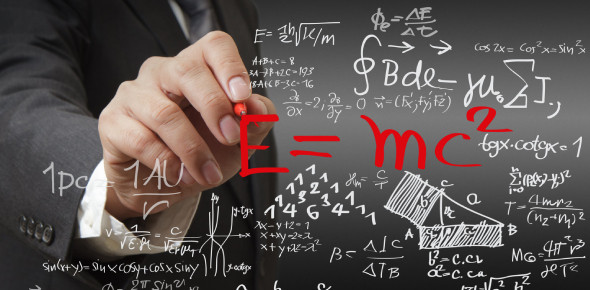# Math Quiz For Primary School Students

Approved & Edited by ProProfs Editorial Team
The editorial team at ProProfs Quizzes consists of a select group of subject experts, trivia writers, and quiz masters who have authored over 10,000 quizzes taken by more than 100 million users. This team includes our in-house seasoned quiz moderators and subject matter experts. Our editorial experts, spread across the world, are rigorously trained using our comprehensive guidelines to ensure that you receive the highest quality quizzes.
| By Hsm2456
H
Hsm2456
Community Contributor
Quizzes Created: 3 | Total Attempts: 20,363
Questions: 10 | Attempts: 19,626SettingsGet ready for a Maths Quiz For Primary School Students. Most people fear mathematics that stems from other people telling them that the subject is meant to be understood by a few students. To be a pro when it comes to mathematics all you need to do is a lot of practice. To get you started, we have prepared the quiz below. Give it a try!

• 1.

### 245+756

• A.

1239

• B.

1001

• C.

8354

• D.

0282

• E.

4857

B. 1001
Explanation
The correct answer is 1001 because when we add 245 and 756, we get a sum of 1001.

Rate this question:

• 2.

1488
• 3.

• A.

6349

• B.

9402

• C.

8644

• D.

9775

• E.

5664

B. 9402
• 4.

• A.

4537

• B.

3948

• C.

5473

• D.

6485

• E.

0988

E. 0988
• 5.

### 5647-4673

• A.

0485

• B.

0475

• C.

0974

• D.

0847

• E.

0465

C. 0974
Explanation
The given expression is a subtraction problem: 5647 - 4673. When subtracting these two numbers, the result is 0974.

Rate this question:

• 6.

### If I had 14 euro and spent 7.36 euro, how much had I left?

• A.

6.39 euro

• B.

5.03 euro

• C.

5.39 euro

• D.

6.64 euro

• E.

6.40 euro

D. 6.64 euro
Explanation
If you had 14 euro and spent 7.36 euro, you would have 14 - 7.36 = 6.64 euro left.

Rate this question:

• 7.

### 19 +345 is equal to 868-34

• A.

Yes

• B.

No

B. No
Explanation
The given statement "19 + 345 is equal to 868 - 34" is incorrect. To verify this, we can calculate both sides of the equation. On the left side, 19 + 345 equals 364. On the right side, 868 - 34 equals 834. Since these two values are not equal, the statement is false, and the correct answer is "no."

Rate this question:

• 8.

### If 100 cents is equal to 1 dollar. 3 dollars are equal to: -

• A.

400 cents

• B.

300 cents

• C.

500 cents

• D.

1000 cents

B. 300 cents
Explanation
If 100 cents is equal to 1 dollar, then 3 dollars would be equal to 300 cents. This is because if 1 dollar is equal to 100 cents, then multiplying both sides of the equation by 3 would give us 3 dollars equaling 300 cents.

Rate this question:

• 9.

### Which one of these is wrong?

• A.

560 is an even number

• B.

459 is an odd number

• C.

Anything that is added into 0 becomes 0

• D.

500 + 550 is equal to 1050

C. Anything that is added into 0 becomes 0
Explanation
The statement "Anything that is added into 0 becomes 0" is incorrect because any number added to 0 will result in the same number. Adding 0 to any number does not change its value.

Rate this question:

• 10.

### 250 + 233 + 517 is equal to:

• A.

1001

• B.

1302

• C.

983

• D.

1000

D. 1000
Explanation
The sum of 250, 233, and 517 is 1000.

Rate this question:

Related TopicsBack to top